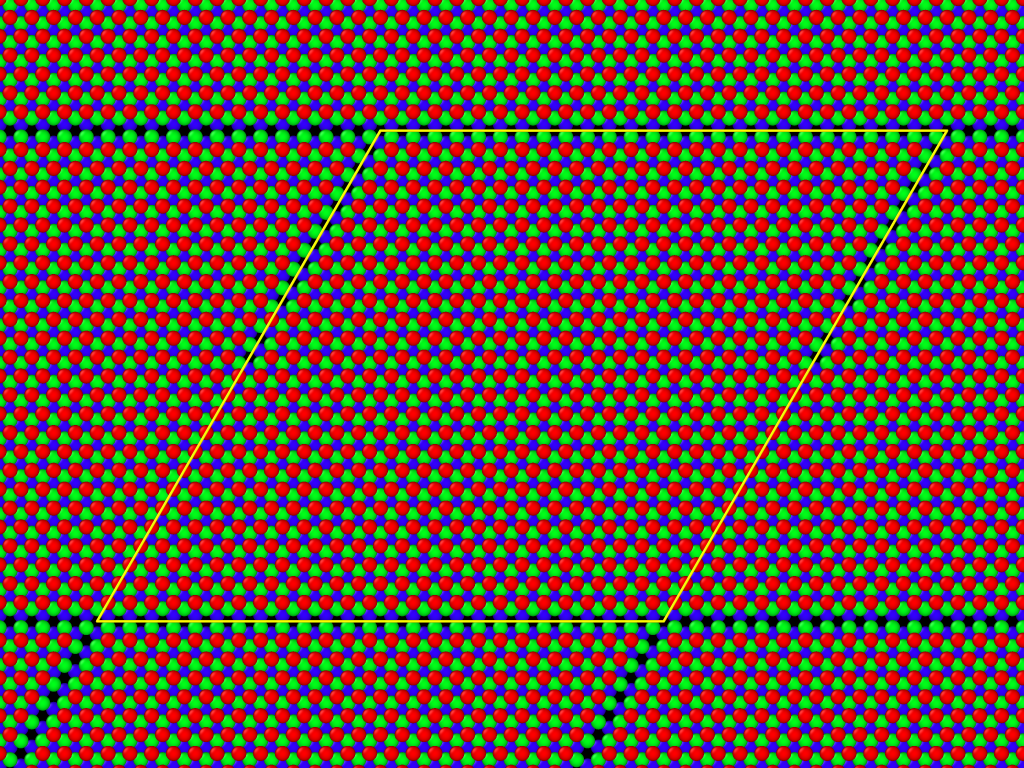# Mistake on the Tersoff/mod webpage

Dear Everyone,

I think there is an erratum on the webpage about the new Tersoff/mod potential (http://lammps.sandia.gov/doc/pair_tersoff_mod.html).
I’ve fitted the Tersoff/mod potential parameters to a certain DFT potential curve using the formulas on the webpage and then I reproduced the potential curve with LAMMPS using the same fitted paramteres. The two potential curves were not the same. That’s why i cheked the original paper about the modified Tersoff potential and found a difference between the original formula and the one on the webpage. The cutoff function should have a PI/2 in the sine not PI. I also cheked the pair_tersoff_mod.cpp file which uses PI/2, so it’s ok, the mistake is only on the webpage. With PI/2 I got the same potential curve as LAMMPS.

Aidan - do you want to check this out with Vitaly (author of MOD extension)?

Steve

After looking at the code and looking at the limits r=R-D, r=R+D, I agree with Szendro. It was a typo in the doc page. I have checked in the correction to the repo:

Sending Eqs/pair_tersoff_mod.jpg
Sending Eqs/pair_tersoff_mod.tex
:
[[email protected]…2929… doc]\$ svn diff -rPREV Eqs/pair_tersoff_mod.tex
:

• \frac{1}{2} - \frac{9}{16} \sin \left( \pi \frac{r-R}{D} \right) - \frac{1}{16} \sin \left( 3\pi \frac{r-R}{D} \right) &
• \frac{1}{2} - \frac{9}{16} \sin \left( \frac{\pi}{2} \frac{r-R}{D} \right) - \frac{1}{16} \sin \left( \frac{3\pi}{2} \frac{r-R}{D} \right) &

Aidan

Dear Szendrő Márton, thank you very much!

You are right, there is an erratum on the webpage about the new
Tersoff/mod potential (http://lammps.sandia.gov/doc/pair_tersoff_mod.html).
The cutoff function must have a PI/2 in the 1st sine and 3PI/2 in the 2nd.
In the near future I will correct the drawing and will mail to Steve.

Regards,
Vitaly

02.10.2013 13:20, Szendrő Márton пишет:

Aidan, thank You a lot!
Now all is OK, I think.

Regards,
Vitaly

02.10.2013 19:04, Thompson, Aidan ???:

Good. You are welcome!

Dear Everyone,

I think there is a serious round-off error when creating triclinic simulation box with a ‘prism’ region.
I’m trying to simulate a multilayer system which consists of several 1 atom thick hexagonal lattices above each other. These layers are parallel with the x-y plane, and i want the simulation to be perfectly periodic in these directions (x-y plane). I made a 60 degree triclinic simulation box, which side’s length are equal, and the size is the integer multiple of the lattice parameter, so there should be no problem with the periodic boundary conditions. However cheking the result atom configuration i found that some random atoms are missing at the boundary (i attached an image of the problem). Checking my log file i set the ylo and yhi parameter of the triclinic box as:

variable ylo equal (-0-2.49415316289918)0.526
variable yhi equal (-0+2.49415316289918)0.526

So clearly the y-length of the simulation cell vector should be 64.8479822353787. However, i think LAMMPS deals with the box dimensions with fewer decimal digits, during run i get the following runtime massage:

Created triclinic box = (-56.16 -32.424 -17.7091) to (18.72 32.424 3.6) with tilt (37.44 0 0)

wich tells me, that the y-length of the created simulation box is rounded up and is 2*32.424 = 64.848. When i visualize the atoms, i measure the same rounded up size, so i guess the dimension values were not rounded up only for output purposes. I think the rounding up is the cause of the missing atoms at the border.
Can someone confirm me with this remark? Is there an elegant way to get rid off the missing atoms problem? I mean changing slightly the simulation cell size in a trial-error way until the atoms are in their correct place is not too elegant in my opinion.Everything internal to LAMMPS is stored in double precision,

so I think you are just seeing the normal C-style rounding

on output. What do you see in the data file if you do a write_data

command, after creating the system? Those box values are

printed at high precision.

Can you post the lines you used to create

the box and the atoms that resulted in missing atoms

at the boundaries?

Steve